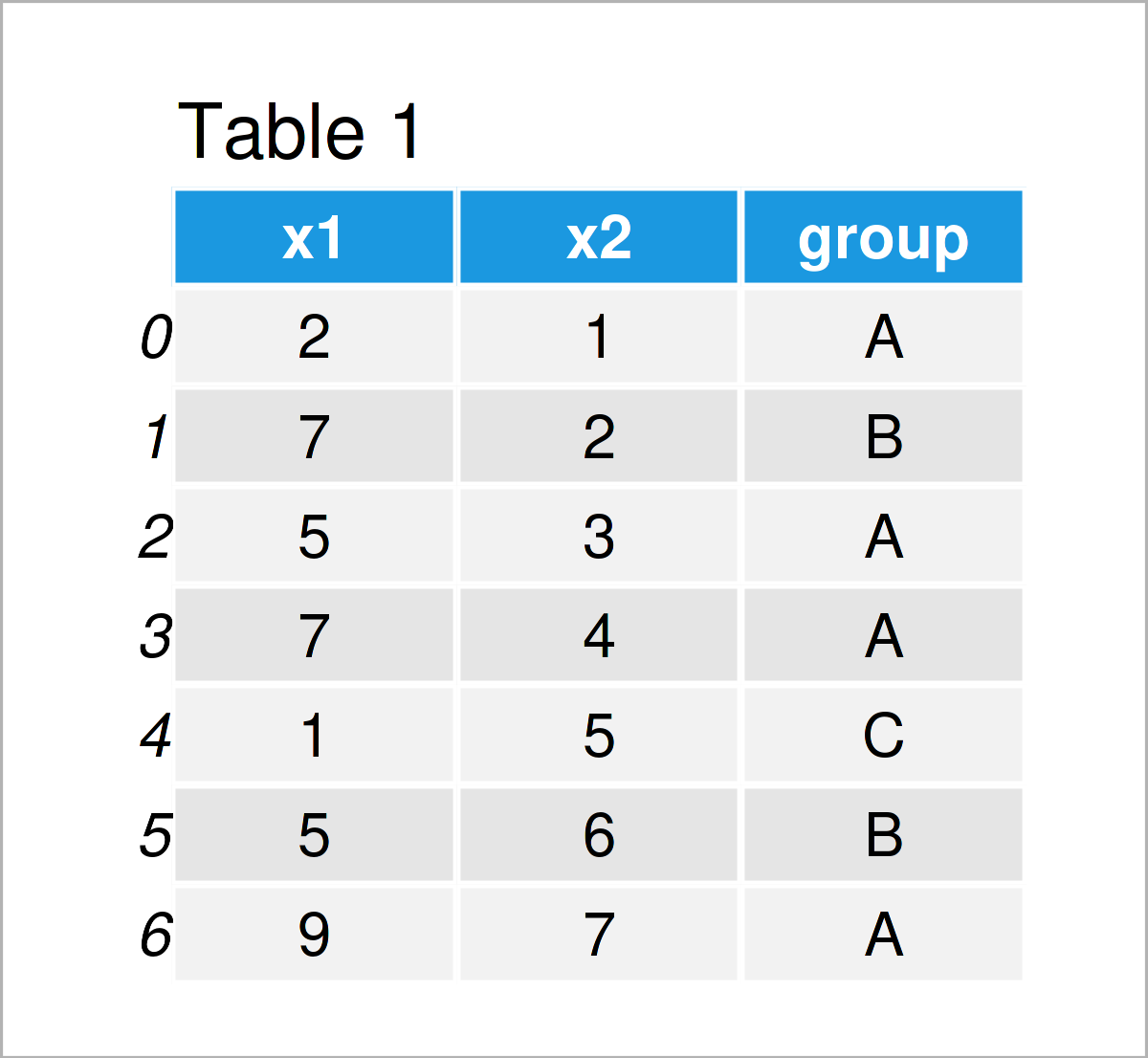# Summary Statistics of pandas DataFrame in Python (4 Examples)

In this article, I’ll illustrate how to calculate descriptive statistics for the columns of a pandas DataFrame in the Python programming language.

The article consists of four examples for the calculation of descriptive statistics for each column of a pandas DataFrame. To be more specific, the content of the article looks like this:

You’re here for the answer, so let’s get straight to the examples…

## Example Data & Software Libraries

In order to use the functions of the pandas library, we first have to load pandas.

`import pandas as pd                               # Load pandas`

As a next step, we’ll also need to define some example data:

```data = pd.DataFrame({'x1':[2, 7, 5, 7, 1, 5, 9],  # Create pandas DataFrame
'x2':range(1, 8),
'group':['A', 'B', 'A', 'A', 'C', 'B', 'A']})
print(data)                                       # Print pandas DataFrame```Have a look at the previous table. It reveals that our example pandas DataFrame has seven rows and three columns.

Let’s compute some summary statistics (or descriptive statistics) for this data set!

## Example 1: Calculate Mean for One Column of pandas DataFrame

This example shows how to calculate descriptive statistics for a single pandas DataFrame column.

More precisely, the following Python code calculates the average of the values in the column x1:

```print(data['x1'].mean())                          # Get mean of one column
# 5.142857142857143```

As you can see based on the previous output, the average of the column x1 is 5.14.

To get this result, we have used the mean function. Note that we could simply exchange the mean function by other functions such as var to get the variance or std to get the standard deviation.

## Example 2: Calculate Mean for All Columns of pandas DataFrame

In this example, I’ll illustrate how to compute summary statistics for multiple variables of a pandas DataFrame.

To accomplish this, we simply can apply a function such as mean to an entire pandas DataFrame:

```print(data.mean(numeric_only = True))             # Get mean of all columns
# x1    5.142857
# x2    4.000000
# dtype: float64```

The previous output shows the mean values for all float columns in our data set (i.e. x1 and x2).

## Example 3: Multiple Summary Statistics for All Columns of pandas DataFrame

So far, we have calculated only one specific metric (i.e. the mean). However, it is also possible to calculate multiple different summary statistics for each column of a pandas DataFrame.

This section shows how to use the describe function to return the count, mean, standard deviation, minimum, 25% quantile, 50% quantile, 75% quantile, and the maximum value in each column.

For this task, we can apply the Python syntax below:

```print(data.describe())                            # Get descriptive statistics of all columns
#              x1        x2
# count  7.000000  7.000000
# mean   5.142857  4.000000
# std    2.853569  2.160247
# min    1.000000  1.000000
# 25%    3.500000  2.500000
# 50%    5.000000  4.000000
# 75%    7.000000  5.500000
# max    9.000000  7.000000```

The previous Python code has returned multiple descriptive statistics for each of the float columns in our data set.

## Example 4: Calculate Mean by Group for All Columns of pandas DataFrame

The syntax below demonstrates how to compute particular summary statistics for the columns of a pandas DataFrame by group.

Consider the Python code below:

```print(data.groupby('group').mean())               # Get mean by group
#          x1    x2
# group
# A      5.75  3.75
# B      6.00  4.00
# C      1.00  5.00```

As you can see, we have calculated the mean values for our two columns x1 and x2 for each group in our data set separately.

## Video & Further Resources

In case you need more info on the Python syntax of this tutorial, you may want to watch the following video on the Statistics Globe YouTube channel. In the video, I’m explaining how to explore data sets using the code of this tutorial in Python.

Please accept YouTube cookies to play this video. By accepting you will be accessing content from YouTube, a service provided by an external third party.If you accept this notice, your choice will be saved and the page will refresh.

In addition, you may have a look at the other tutorials on my homepage:

To summarize: At this point you should know how to get summary statistics and explore all the columns of a pandas DataFrame in Python programming. If you have further questions, let me know in the comments section below. Furthermore, don’t forget to subscribe to my email newsletter to get updates on new articles.

Subscribe to the Statistics Globe Newsletter